Arches

Abs¤luteC¤mplementC¤mpleteComsistentInterrelati¤nshipUmcærtain

Here we use standard probability equations to produce curves shown.

 Our approach follows a line of classical reasoning like this: Assume a probability that no two physical (i.e., actual) comtexts are comsistent out of a group of 'n' comtexts in 't' total possible comtexts is, S(n,t)=t!/((t-n)! * tn). Then we assume a probability that two or more physical comtexts are more c¤mplete out of a group of 'n' comtexts is, P(n,t) = 1 - S(n,t). We approximated our calculation of S(n,t) using (1-n/(2*d))n-1 Classical Problematics (need wingdings font for our fickle quantum fingers :):Analyticity (determinism, induction, cause-effect, homogeneous time or independent variable as change, axiom of independence (a strict derivation of Aristotle's 3rd syllogistic 'law,' claiming excluded-middle 'independence' of classical objects), et al.) - As you can see in our graph above and our classical equations, a classical assumption of analyticity is inherent. Our classical equation is continuous. But is reality analytic? N¤! Reality is quantal. Do our continuous, analytic graphs of our probability functions for classical consistency and completeness depict quantal reality? N¤!Localability, isolability/individuicity, separability, and reducibility (lisr) - A classical assumption of lisr is inherent in classical reasoning. Each classical context is lisr. Each classical context adheres Aristotelian syllogisms, especially objective, excluded-middles among all classical contexts. But is reality lisr? N¤! Reality is quantum c¤hesive. Do our continuous, analytic graphs of our probability functions for classical consistency and completeness depict quantum c¤hesive reality? N¤!Ideal mathematical integer constancy - A classical assumption that an ideal logical/physical constant, one (1), exists. A classical assumption that ideal logical/physical manipulations of one (1) exist: 1/1 iso 1 1*1 iso 1 1+1 iso 2 (inference of induction and counting) 1-1 iso 0 (inherent definition of zero) 1 = 1 iso classical ideal identity d1/dt = 0 (1st derivative of a 'constant' is zero; assumes homogeneous time) (1-1)/(1-1) iso undefined (Isn't this amazing?) But does reality generate or manifest physical integer constants? N¤! All quantum n¤mbærs are umcærtain! N¤ tw¤ quantum n¤mbærs are identical! In general it is n¤t p¤ssible f¤r tw¤ quantum n¤mbærs t¤ be physically and thus l¤gically identical. Do our continuous, analytic graphs of our probability functions for classical consistency and completeness depict quantum n¤mbær umcærtainty? N¤! (I.e., use of '1-P.') So, reader, you can see how classical concepts, especially classical mathematical concepts, impede any real and natural understanding of quantum reality. N¤ mathematician ever shows '1' as a quantum umcærtain comcept. (Using n¤vel memes presented here we can d¤ that.) F¤r ¤ur purp¤ses here, let's assume that ¤ur least values f¤r comsistency and c¤mpleteness in ¤ur n¤nclassical, quantum reality are individual quanta. Our curves d¤ n¤t sh¤w that; h¤wever, we can imagine their classical zero asymptotes as reality's minimum Planck quantum, i.e., quantum_2•quantum_•h.

Reality ¤ffers unlimited quantum comtexts. We only show 100 of 1000 modeled positive entropy possibilities.

F¤r a single p¤sitive entr¤py comtext, we assume comsistency, Cn, is maximum, and c¤mpleteness, Cm, is minimum. F¤r an unlimited number ¤f comtexts, we assume comsistency, Cn, is minimum, and c¤mpleteness, Cm, is maximum.

Our comjecture is that a minimum actual (i.e., p¤sentr¤py) comtext is a single, Planck quanton representing a single quantum ¤f latched energy ¤r mass equivalent which physicists usually model as e=hf or e=hv
(f, v, are frequency; h is Planck's constant; and e is energy),
and which we ch¤¤se t¤ m¤del as e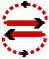hv. (with frequency v as: quantum_1 timæs quantum_2, where: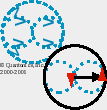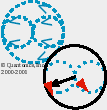quantum_1, and quantum_2)We classically assume that PCm = 1-PCn.(re: our plotted equations above)

Thus, Classical Thing-king Methods tell us that e can be arbitrarily small and thus can approach zero analytically.

We quantumly/Quantonically assume that PCmquantum_1-PCn
(with Planck quantum abscissa asympt¤tes)

See our graph above.

We quantumly assume that ¤ur smallest, inc¤mplete comtext is ¤ne smallest Planck quantum.
We quantumly assume that ¤ur smallest comsistent comtext is ¤ne smallest Planck quantum.
We quantumly assume that ¤ur largest, c¤mplete comtext is infinite comtexts ¤f Planck quanta.
We quantumly assume that ¤ur largest incomsistent comtext is infinite comtexts ¤f Planck quanta.
(comtexts ab¤ve are actualized quantons)

Thus, Quantonic/Quantum Think-king M¤des tell us that e can be n¤ smaller than hv.

We can imagine ¤ur least quantum ¤f b¤th acti¤n and certainty quanton as equantum_2h.

We warned you about quantum_1, quantum_2, etc. What about quantum? (7Dec2001 - Doug.)

Using ¤ur Quantonic n¤tati¤n we see:

quanton(preferential_is¤flux,a_Planck_quantum).

Using ¤ur Quantonics f¤nt symb¤ls we can sh¤w ¤ur quanton like this:

 quanton(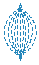,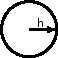)

T¤ see an applied use ¤f ¤ur is¤flux symb¤l, see ¤ur quantum spin c¤rrelati¤n graphic.

Using pure Quantonics symb¤l¤gy we can sh¤w ¤ur least quantum pragma like this:T¤ f¤ll¤w its ¤nt¤l¤gy, see ¤ur Quanton Spin Interrelati¤nships Emergence artw¤rk. This ¤nt¤l¤gy is useful. It will help us t¤ further inn¤vate ¤ur zer¤entr¤py and negentr¤py Quantonic percepts ¤f quantum reality.

When ¤ne comsiders Planck's least quanta ¤f acti¤n as the basis f¤r Heisenberg's uncertainty principle, and further comsiders h¤w reality builds actuality fr¤m quanta, ¤ur Quantonics comjecture ¤f abs¤luteness as a quantum umcærtainty interrelati¤nship twixt comsistency and c¤mpleteness is n¤t s¤ far fetched.

D¤ug.
25Aug2000

To contact Quantonics write to or call:

Doug Renselle
Quantonics, Inc.
Suite 18 #368 1950 East Greyhound Pass
Carmel, INdiana 46033-7730
USA
1-317-THOUGHT

©Quantonics, Inc., 2000-2022 — Rev. 10Sep2012  PDR — Created 25Aug2000  PDR
(30Aug2000 rev - Extend classical problematics.)
(3Sep2000 rev - Change "derivatives of 1" to "manipulations of 1.")
(9Oct2000 rev - Add quantum_1 and quantum_2 to e as 2
ph calculation.)
(14Nov2000 rev - Change Classical 'Modes' to Classical 'Methods.')
(14Nov2000 rev - Change '1' to 'quantum_1' in our quantum version of PCm = 1-PCn, a classical probability equation.)
(14Nov2000 rev - Ditto 'quantum_2.' Add link to our quantum spin correlation graphic.)
(27Nov2000 rev - Add anchor to Quantum Integers, 1 and 2.)
(10Apr2001 rev - Change all quantum comtextual 'o' characters to '¤' characters. Add explanatory link(s).)
(10Apr2001 rev - Change all quantum comtextual 'con' prefixes to 'com,' except in uncertainty graphic.)
(10Jun2001 rev - Correct typos, e.g., 'Problemetics.')